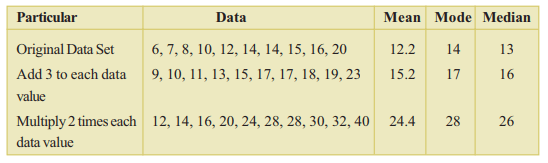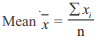# AP Board Class 9 Maths Chapter 9 Statistics

Statistics is one of the important chapters for Andhra Pradesh Board Class 9 Maths students. Several questions are usually asked in the exams and students have to be thorough with the topics. As such, in AP Board Class 9 Maths Chapter 9 Statistics, students will basically understand topics related to data handling and study the process of extraction of meaning from the data. Some main topics covered are given below:

• Collection Of Data
• Presentation Of Data
• Measures Of Central Tendency (including Mean, Median and Mode)
• Deviation in Values of Central Tendency

## What is Statistics?

Statistics is all about the collection, presentation, analysis, and interpretation of data. Statistics is used primarily to collect data with some purpose or determine the numerical data.

### Collection of Data

Data can be collected in several ways and with different motives. A researcher can collect data with a definite or clear objective in mind. This type of data is known as primary data. There is also what is known as secondary data which is the information gathered directly from a source. Here the data is already recorded and present.

### Presentation of Data

After the data is collected it has to be presented in a meaningful manner depending on the different situations. Presentation of data is done in the form of tabular, textual or graphical. Presenting data in these various forms will help in simplifying and condensing data. Further, it also allows the observer to take a quick glance at certain important features.

### Measures of Central Tendency

Measures of central tendency are one of the most basic and useful statistical functions. Measures of central tendency are also sometimes referred to as summary statistics. However, if we look at the definition, a measure of central tendency is a single measure or value that represents a whole set of data by identifying the centre point. In simple words, measures of central tendency are numbers that help to know what is average within a distribution of data. There are three types of measures of central tendency, namely, which are;

• Mean: The sum observation of a data, which is divided by the number of observations gives the mean.
• Median: It is the middle observation of a given raw data when it is arranged in either ascending or descending order.
• Mode: An observation with the maximum frequency is known as a mode.

### Deviation in Values of Central Tendency

This topic basically covers the impact of addition and multiplication of values on the measures of central tendency.Students can refer to some chapter questions along with their solutions below;

Question 1: Write down three examples of data that can be collected in everyday life.

Solution: The three examples are;

(i) The number of boys and girls in a class.

(ii) Mobile phone bills for the last six months.

(iii) Daily household expenses

Question 2: If the mean of 9, 11, 13, 17, P and 20 is 15, find the value of P.

Solution: We know,$15=\frac{9+11+13+P+17+20}{6}$

90 = 70 + P

Therefore, P = 20

Students can stay connected to BYJU’s to know more about AP Board and access various study materials, including syllabus, exam timetable and more.